### Effect of harmonics on Performance of 3-ph Induction Motor

The induction motor performance is affected by the harmonics in the time variation of the impressed voltage. But its effect on the performance of the motor is not predominant hence it is not considered here.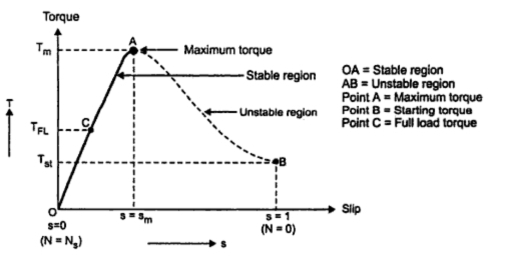Fig. 1 Torque speed characteristics

The torque-slip characteristics as shown in Fig.1 is obtained when the space distribution of flux wave along the air gap periphery is sinusoidal. But the air gap flux is not purely sinusoidal as it contains odd harmonics (5th, 7th, 11th etc). Hence at low speeds, the torque-slip characteristic is not smooth. The distribution of stator winding and variation of air gap reluctance due to stator and rotor slots are main causes of air gap flux harmonics.
The harmonics caused due to variation of air gap reluctance are called tooth or slot harmonics . Due to these harmonics produced in air gap flux, unwanted torque are developed along with vibration and noise.
Now eventhough stator currents are sinusoidal, the stator m.m.f. is not sinusoidal as stator winding has the number of slots not more than 3 to 4 per phase. If carry out analysis of stator m.m.f. with the help of Fourier series it can be seen that in addition to fundamental wave it contains odd harmonics m.m.f. waves.
The third harmonic flux waves produced by each of the three phases neutralize each other as it differs in time phase by 120o. Thus air gap flux does not contain third harmonics and its multiplies. The fundamental mmf wave produces flux which rotates at synchronous speed which given as ns = 2f1/P rps where f1 is supply frequency and P is number of poles. Similarly fifth harmonic mmf wave produces flux which rotates at 2f1/5P = ns/5 rps and in direction opposite to the fundamental mmf wave. The seven harmonic mmf produces flux which rotates at ns/7 rps and in the direction of fundamental m.m.f. wave.
Thus it can be seen that harmonic m.m.f. wave produces flux which rotates at 1/K times the fundamental speed and in the direction of fundamental wave if K = 6m + 1 and in the reversed direction if K = 6m - 1 where m is any integer. The most important and predominant harmonics whose effects must be studied are 5th and 7th harmonics.
The electromagnetic torque that is developed in the induction motor is because of zero relative speed between stator and rotor fields. This fact can be explained as follows :
When rotor is revolving in the same direction of rotation as the stator field, the frequency of rotor currents is sf1 and the rotor field produced will have speed of sns rpm with respect to rotor in the forward direction. But there is mechanical rotation of rotor at n rpm which is superimposed on this. The speed of rotor field in space is thus given by sum of these speeds
sns + n = sns +  ns(1-s) = ns
The stator and rotor fields are thus stationary with respect to each other which produces a steady torque maintaining the rotation. This torque existing at any mechanical speed n other than synchronous speed is called synchronous torque.
The fifth harmonic field rotates at ns/5 rps and in a direction opposite to direction of rotor. Therefore slip of rotor with respect to fifth harmonic field speed is

Here -ns/5 represents fifth harmonic field rotating opposite to the rotor. The frequency of rotor currents induced by fifth harmonic rotating field is
f2 fifth harmonic  = s5 x Stator frequency
= (6 -5s) x f1
Now speed of fifth harmonic rotor field with respect to rotor is given by

Now, speed of fifth harmonic rotor field with respect to stator

Negative sign is used before ns/5 (6 - 5s) which indicates 5th harmonic field rotates opposite to rotor movement. Thus it can be seen that speed of fifth harmonic stator field and rotor field is equal and relative speed between the two is zero. Thus it produces 5th harmonic induction motor torque similar to torque produced by fundamental component.
Similar analysis can be made on 7th harmonic to show 7th harmonic torque produced similar to fundamental one. Thus each space harmonic can be considered to produces its own asynchronous torque. The induction motor can be considered as equivalent to number of induction motors in series having poles equal to number of harmonics multiplied by number of poles. The torque produced by fundamental component and the harmonic are shown in the Fig. 2.Fig. 2   Presence of harmonics

1.1 Crawling
As fifth harmonic field rotates opposite to the rotor rotation, the torque produced by fifth harmonic opposes fundamental torque and it acts as braking torque on motor. The seventh harmonic field rotates in the direction of rotor rotation, the torque produced by seventh harmonic aids the fundamental torque. The resultant torque is shown in the Fig. 2 which shows the addition of fundamental, fifth harmonic and seventh harmonic torque. The fifth harmonic torque is zero at -ns/5 rps while seventh harmonic torque is zero at +ns/7.
There are two dips which can be seen in the resultant torque, one is near the slip 1.2 and other near slip 6/7. The slip near s =6/7 is more important as torque here decreases with increase in speed. The load torque is shown in figure. The rotor will run at ns/7 with X as the operating point. Thus stable operation is obtained near sub-synchronous speed ns/7. This is called crawling or synchronous crawling. Due to crawling there is much higher stator current accompanied by noise and vibration. The torque obtained from induction motor here is called synchronous called.
When two harmonic fluxes of same order one because of stator and the rotor because of rotor interact with each other at one particular speed and produces harmonic synchronous torque just like that produced in synchronous motor. These torques are caused by tooth harmonics. The stable operation at synchronous speed caused by slot harmonics is called synchronous crawling which is associated with vibration and noise.
1.2 Cogging
A special behaviour is shown by squirrel cage induction motor during starting for certain combinations of number of stator and rotor slots. If number of stator slots S1 are equal to number of rotor slots S2 or integral multiple of rotor slots S2 then variation of reluctance as a function of space will have pronounced effect producing strong forces than the accelerating torque. Due to this motor fails to start. This phenomenon is called cogging. Such combination of stator and rotor slots should be avoided while designing the motor.
Let the slots of stator and rotor be 24. The stator-slotting produces its tooth harmonics of order (2S1/P) ±1 whereas the rotor-slotting produces its tooth harmonics of order (2S1/P) ±1 where S1 and S2 are number of stator and rotor slots. The plus sign refers to the harmonic field rotation in the direction of rotor.
Here S1 = S2 so stator and rotor slot harmonics are same and given by,
Let                  P = 4
(2x24/ 4) ± 1 = 11 or 23
The harmonics of order 11 produce backward rotating field for both stator and rotor. The harmonics of order 13 produces forward rotating field.
The two harmonics fields of same order say 11th harmonic would be stationary with respect to each other only when
nr - (ns-nr / 11) = -ns/11
nr = 0
As the harmonic field due to 11th  harmonic rotates backward with respect to stator hence negative sign is used for ns/11.
Similarly, for 13th  harmonic produced by stator and rotor would be stationary with respect to each other when
= (ns-nr / 13) + nr = ns/13
nr = 0
Hence it can be seen that harmonic synchronous torque is produced at zero rotor speed. The 11th  and 13th  harmonic fields produced by stator and rotor and stationary with respect to each other. The harmonic synchronous torque is produced at zero rotor speed and the motor will remain at rest. This is called cogging. The torque speed characteristic with harmonic synchronous torque as ns/7 is shown in the Fig.3.Fig. 2   Cogging

The stator slot harmonics of order (2S1/P) ±1 may interact with rotor slot harmonics of order (2S2/P) ± 1 to develope the harmonic synchronous torques.
(2S1/P) + 1 = (2S2/P) + 1
S1 = S2
And           (2S1/P) -1 = (2S2/P) + 1
S1-S2 = P
It can be thus seen that if S1 = S2 or S1-S2 = P then cogging will be definately observed in the induction motor.
The cogging and crawling is not predominately in slip ring induction motor as these motors are started with higher starting torques with external resistance in rotor circuit.
The crawling effect can be reduced by taking proper car during the design. Still if crawling is observed then it can be overcome by applying a sudden external torque to the driven load in the direction of rotor. If there is reduction in supply voltage then torque also decreases (Tα V12). Hence asynchronous crawling may be observed which is absent under rated voltage conditions. Thus asynchronous torques can not be avoided but can be reduced by proper choice of coil span and by skewing the stator or rotor slots.
Key Point : The synchronous harmonics torques can be totally eliminated by proper combination of stator and rotor slots.
Hence it can be seen that harmonic synchronous torque is produced at zero rotor speed. The 11th and 13th  harmonic fields produced by stator and rotor and stationary with respect to each other. The harmonic synchronous torque is produced at zero rotor speed and the motor will remain at rest. This is called cogging. The torque speed characteristics with harmonic synchronous torque as ns/7 is shown in the Fig..3.
The stator slot harmonics of order 2S1/P ±1 may interact with rotor slot harmonics of order 2S1/P ±1 to develope the harmonic synchronous torques.
2S1/P +1 = 2S2/P +1
S1 = S2
And          2S1/p -1 = 2S2/P +1
S1- S1= P
It can be thus seen that if S1= S2 or S1 - S2 = P then cogging will be definately observed in the induction motor.
The cogging and crawling is not predominately in slip ring induction motor as these motors are started with higher starting torques with external resistance in rotor circuit.
The crawling effect can be reduced by taking proper care during the design. Still if crawling is observed then it can be overcome by applying a sudden external torque to the driven load in the direction of rotor. If there is reduction of in supply voltage then torque also decreases (Tα V12). Hence asynchronous crawling may observed which is absent under rated voltage conditions. Thus asynchronous torques can not be avoided but can be reduced by proper choice of coil span and by skewing the stator or rotor slots.
Key Point : The synchronous harmonics torques can be totally eliminated by proper combination of stator and rotor slots.

1.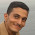2.great explanation ! & thanks a lot for sharing !

3.i'm sorry, could you explain why the seventh harmonic frequency is 1/7 of the original freq and not 7 times f1 ?

1.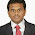THE REASON IS IT IS SPACE HARMONICS THAT IS DUE NON SINUSOIDAL VARIATION OF FLUX DENSITY.IN THIS CASE YOU HAVE TO MULTIPLY POLES=(HARMONICS NUMBER * NO OF FUNDAMENTAL POLES).DO NOT REPLACE FREQUENCY=5*FREQUENCY.THAT IS TIME HARMONICS i.e harmonics in supply.

4.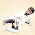hai sir your site is really awesome.Inspired by your site I have started a blog Electrical Engineering Info but could not be successful.Can you help me sir

5.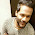Great Help to whom who want to study self !!

6.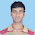7.Good article...

8.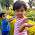9.(2x24/ 4) ± 1 = 11 or 23
2x24/ 4) ± 1 = 11 or 13*